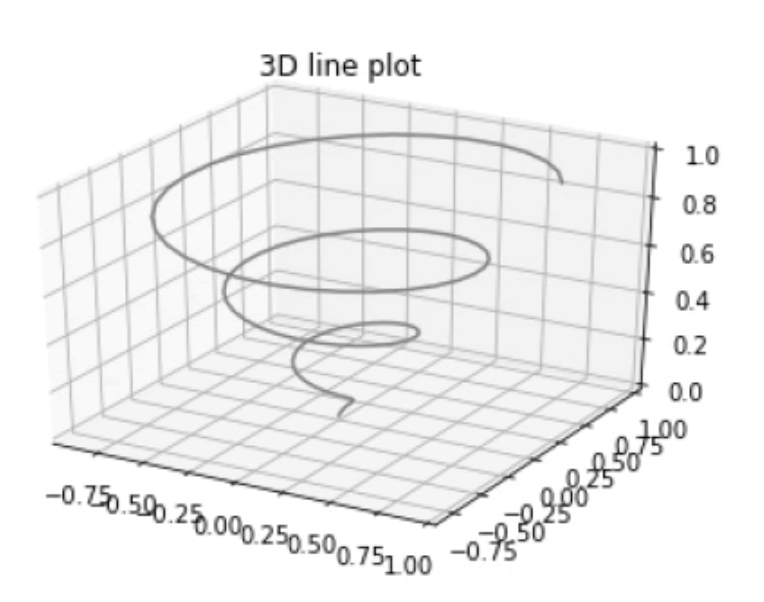# Python Matplotlib 3D绘图详解(汇总)

最初开发的 Matplotlib，仅支持绘制 2d 图形，后来随着版本的不断更新， Matplotlib 在二维绘图的基础上，构建了一部分较为实用的 3D 绘图程序包，比如 mpl_toolkits.mplot3d，通过调用该程序包一些接口可以绘制 3D散点图、3D曲面图、3D线框图等

mpl_toolkits 是 Matplotlib 的绘图工具包。

## 第一个三维绘图程序

下面编写第一个三维绘图程序。

首先创建一个三维绘图区域， plt.axes() 函数提供了一个参数projection，将其参数值设置为 "3d"。如下所示：

``````#导入三维工具包mplot3d
from mpl_toolkits import mplot3d
import numpy as np
import matplotlib.pyplot as plt
fig = plt.figure()
#创建3d绘图区域
ax = plt.axes(projection='3d')``````

有了三维绘图区域，接下来就要构建 3d 图像，如下所示：

``````#从三个维度构建
z = np.linspace(0, 1, 100)
x = z * np.sin(20 * z)
y = z * np.cos(20 * z)``````

最后调用 plot3D() 方法绘制 3d 图形，代码如下：

``````#调用 ax.plot3D创建三维线图
ax.plot3D(x, y, z, 'gray')
ax.set_title('3D line plot')
plt.show()``````

完整程序如下所示：

``````from mpl_toolkits import mplot3d
import numpy as np
import matplotlib.pyplot as plt
fig = plt.figure()
#从三个维度构建
z = np.linspace(0, 1, 100)
x = z * np.sin(20 * z)
y = z * np.cos(20 * z)
#调用 ax.plot3D创建三维线图
ax.plot3D(x, y, z, 'gray')
ax.set_title('3D line plot')
plt.show()``````

输出结果如下所示：上述代码中的 ax.plot3D() 函数可以绘制各种三维图形，这些三维图都要根据(x，y，z)三元组类来创建。

## 3D散点图

通过 ax.scatter3D() 函数可以绘制 3D 散点图，示例代码如下：

``````from mpl_toolkits import mplot3d
import numpy as np
import matplotlib.pyplot as plt
fig = plt.figure()
#创建绘图区域
ax = plt.axes(projection='3d')
#构建xyz
z = np.linspace(0, 1, 100)
x = z * np.sin(20 * z)
y = z * np.cos(20 * z)
c = x + y
ax.scatter3D(x, y, z, c=c)
ax.set_title('3d Scatter plot')
plt.show()``````

输出结果图：